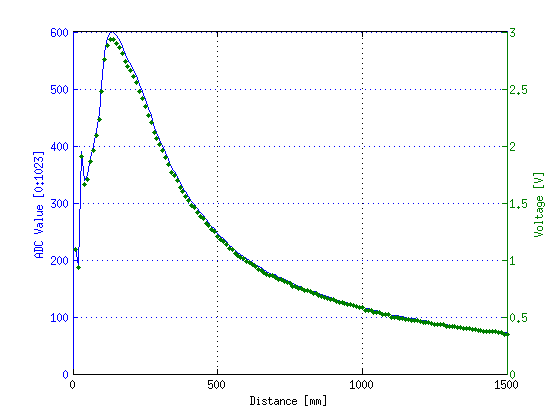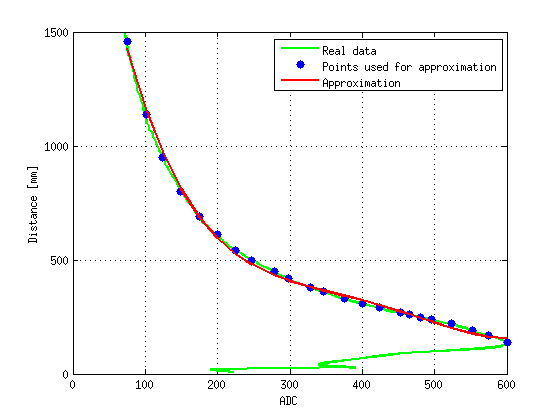Do me a favor, take a few seconds to have a look at my last project.

Thank you, Lulu

# Arduino based accurate distance measurement for Sharp GP2Y0A02YK

The aim of this post is to explain how to convert voltage from Sharp ranging sensor into an accurate distance. Experiments have been performed with Sharp GP2Y0A02YK distance sensors. The range of this triangulation-based sensors is from 20cm to 150cm.

## Measurement campaign

An accurate measurement experimental campaign has been performed. Range has been measured with a step of 10mm. The following graph show the behavior of the GP2Y0A02YK sensor according to the distance (ADC value vs distance). As it is explained in the sensor’s specifications the sensor is not suited for measurements below 20cm due to ambiguity.## Polynomial approximation

Thanks to the Matlab polyfit fonction, the curv has been approximated with a fourth degree polynomial. The approximation covers the range from 14cm to 150cm. The following figure shows the result:## Results

The approximation for the GP2Y0A02YK is given by the following equation. $$ADC$$ is the raw value returned by the Arduino analogRead() function in range from 0 to 1024 (10 bits analog to digital converter).

$$Distance = 2583.711122992086 \\ - 20.197897855471.ADC \\ + 0.071746539329.ADC^2 \\ - 0.000115854182.ADC^3 \\ + 0.000000068590.ADC^4$$

Based on this result, a C++ function can easily be written in order to compute the distance:

/*!
\brief  make a distance measurement with a Sharp GP2Y0A02YK sensor
\return the measured distance in mm

*/
float get_Sharp_GP2Y0A02YK_Distance(int PinID)
{
// Read analog to digital converter value

// Convert in millimeters and return distance
return  2583.711122992086
}